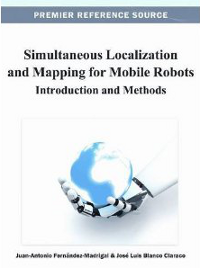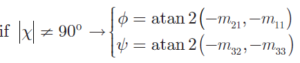# SLAM Book (2012)

Title: Simultaneous Localization and Mapping for Mobile Robots: Introduction and Methods
Authors: Fernández-Madrigal, J.A. and Blanco, J.L.
Release date: 2012
ISBN: 978-1466621046
Pages: 497Available in:

Description

As mobile robots become more common in general knowledge and practices, as opposed to simply in research labs, there is an increased need for the introduction and methods to Simultaneous Localization and Mapping (SLAM) and its techniques and concepts related to robotics.
Simultaneous Localization and Mapping for Mobile Robots: Introduction and Methods investigates the complexities of the theory of probabilistic localization and mapping of mobile robots as well as providing the most current and concrete developments. This reference source aims to be useful for practitioners, graduate and postgraduate students, and active researchers alike.

## Erratum (last update: Feb 2017)

 Errata location Says… Should say… Page 200, eq. (20)Remove all four minus signs. Page 405 Equations fpi(a,p) and its Jacobians Corrected versions are in section 4.2 of this techical report.

# Summary of contents

Part I: The Foundations of Mobile Robot Localization and Mapping

CHAPTER 1: Introduction

CHAPTER 2: Robotic Bases

CHAPTER 3: Probabilistic Bases

CHAPTER 4: Statistical Bases

Part II: Mobile Robot Localization

CHAPTER 5: Robot Motion Models

CHAPTER 6: Sensor Models

CHAPTER 7: Mobile Robot Localization with Recursive Bayesian Filters

Part III: Mapping the Environment of Mobile Robots

CHAPTER 8: Maps for Mobile Robots: Types and Construction

CHAPTER 9: The Bayesian Approach to SLAM

Appendices

APPENDIX I: Common SE(2) and SE(3) Geometric Operations

APPENDIX II: Resampling Algorithms

APPENDIX III: Generation of Pseudo-Random Numbers

APPENDIX IV: Manifold Maps for SO(n) and SE(n)

APPENDIX V: Basic Calculus and Algebra Concepts

Foreword (by Gabe Sibley)

Preface

Acknowledgments

Part I: The Foundations of Mobile Robot Localization and Mapping

CHAPTER 1: Introduction

1.1 OVERVIEW

1.2 TAXONOMIES FOR THE PROBLEMS

The Representation of Spatial Knowledge

The Structure and Dynamics of the Environment

The Sensory Apparatus of the Robot

The Motor Apparatus of the Robot

The Previous Knowledge

1.3 HISTORICAL OVERVIEW

1.4 ORGANIZATION OF THE BOOK

REFERENCES

CHAPTER 2: Robotic Bases

2.1 INTRODUCTION

2.2 TURNING MACHINES INTO ROBOTS: ACTUATORS

Legged Robots

Flying Robots

Submarine Robots

Wheeled Robots

2.3 HOW DOES THE WORLD LOOK TO A ROBOT? SENSORS

2.4 PROPRIOCEPTIVE SENSORS: INERTIAL SENSORS

2.5 EXTEROCEPTIVE SENSORS (I): CONTACT AND VERY SHORT-RANGE SENSORS

2.6 EXTEROCEPTIVE SENSORS (II): SINGLE-DIRECTION RANGEFINDERS

Triangulation-Based Proximity Sensors

Pulse-Signal Time of Flight (P-ToF)

Continuous-Wave Time of Flight (C-ToF)

Final Remarks

2.7 EXTEROCEPTIVE SENSORS (III): TWO-DIMENSIONAL RANGEFINDERS

2.8 EXTEROCEPTIVE SENSORS (IV): THREE-DIMENSIONAL RANGE SENSORS

2.9 EXTEROCEPTIVE SENSORS (V): RANGE-ONLY SENSORS

2.10 EXTEROCEPTIVE SENSORS (VI): IMAGING SENSORS

2.11 EXTEROCEPTIVE SENSORS (VII): AIR ANALYSIS SENSORS

2.12 ENVIRONMENTAL SENSORS: ABSOLUTE POSITIONING DEVICES

2.13 ENERGY SUPPLY

REFERENCES

CHAPTER 3: Probabilistic Bases

3.1 INTRODUCTION

3.2 HISTORICAL OVERVIEW

3.3 PROBABILITY SPACES

3.4 RANDOM VARIABLES

3.5 THE SHAPE OF UNCERTAINTY

The Shape of Uncertainty of Discrete R.V.s

The Shape of Uncertainty of Continuous R.V.s

The Shape of Uncertainty of Any R.V. The Likelihood

3.6 SUMMARIZING UNCERTAINTY

Moments of a R.V.

Information and Entropy of a R.V.

3.7 MULTIVARIATE PROBABILITY

Joint Probability and Marginalization

Mutual Independence and Covariances

3.8 TRANSFORMING RANDOM VARIABLES

Preliminaries

Sum of Two Continuous, Independent R.V.s

Linear Combination of Continuous, Independent R.V.s

Product and Division of Continuous, Independent R.V.s

Linear Transformation of Continuous, Unidimensional R.V.s

Linear Transformation of Multivariate R.V.s

The Especial Case of the Chi-Squared Distribution

Approximating Arbitrary Transformations

3.9 CONDITIONAL PROBABILITY

3.10 GRAPHICAL MODELS

Definitions and Taxonomy

Factorizations

Learning and Inference with Bayesian Networks

Conditional Independence in BNs and D-Separation

Marginal Distributions

The Graph of Correlations

REFERENCES

CHAPTER 4: Statistical Bases

4.1 INTRODUCTION

4.2 IN BETWEEN PROBABILITY AND STATISTICS

Almost Sure Convergence or Convergence with Probability One

Convergence in Probability or in Measure

Convergence in Distribution

Convergence in Norm or in Q-Norm

Probabilistic Convergence and the Limit Laws

4.3 ESTIMATORS

4.4 PROPERTIES OF ESTIMATORS (I): USE OF THE SAMPLE

Completeness

Sufficiency

Robustness

4.5 PROPERTIES OF ESTIMATORS (II): CONVERGENCE TO THE ACTUAL VALUE(S)

Consistency

Biasedness

Risk

4.6 PROPERTIES OF ESTIMATORS (III): UNCERTAINTY (VARIANCE) OF THE ESTIMATOR

Minimum Variance

Efficiency

4.7 CONSTRUCTING ESTIMATORS (I): CLASSICAL ESTIMATORS

Efficient Estimators

Minimum Variance, Unbiased Estimators

Best Linear Unbiased Estimators

Maximum Likelihood Estimators

Least Squares Estimators

Estimators Constructed with the Method of Moments

4.8 CONSTRUCTING ESTIMATORS (II): BAYESIAN ESTIMATORS

Minimum Mean Squared Error Estimator (MMSE)

Maximum A Posteriori Estimator (MAP)

Median Estimator (MED)

4.9 ESTIMATING DYNAMIC PROCESSES

REFERENCES

Part II: Mobile Robot Localization

CHAPTER 5: Robot Motion Models

5.1 INTRODUCTION

5.2 CONSTANT VELOCITY MODEL

Kinematic Equations

Probabilistic Motion Model

Application Example

Extension to the 3D Case

5.3 HOLONOMIC MODEL WITH A DIRECTION AND A DISTANCE

Kinematic Equations

Probabilistic Motion Model

5.4 NON-HOLONOMIC MODEL WITH TWO WHEEL ENCODERS

Kinematic Equations

Probabilistic Motion Model

5.5 NON-HOLONOMIC MODEL WITH ONE ANGULAR AND ONE WHEEL ENCODER

Kinematic Equations

Probabilistic Motion Model

5.6 A BLACK-BOX UNCERTAINTY MODEL FOR COMMERCIAL ROBOTS

Kinematic Equations

Probabilistic Motion Model

5.7 AN ALTERNATIVE MODEL: THE NO-MOTION MOTION MODEL

Kinematic Equations

Probabilistic Motion Model

5.8 IMPROVEMENTS OF THE BASIC KINEMATIC MODELS

REFERENCES

CHAPTER 6: Sensor Models

6.1 INTRODUCTION

6.2 THE BEAM MODEL AND RAY-CASTING

6.3 FEATURE SENSORS (I): PROBABILISTIC MODELS

6.4 FEATURE SENSORS (II): DATA ASSOCIATION

The Nearest Neighbor DA Algorithm (NN)

The Joint Compatibility Branch and Bound Algorithm (JCBB)

Mahalanobis Distance vs. Matching Likelihood

6.5 “MAP” SENSORS

Grid Map Matching

Point Map Matching

REFERENCES

CHAPTER 7: Mobile Robot Localization with Recursive Bayesian Filters

7.1 INTRODUCTION

7.2 PARAMETRIC FILTERS FOR LOCALIZATION

The Kalman Filter (KF)

The Extended Kalman Filter (EKF)

The Unscented Transform and the Unscented Kalman Filter (UKF)

7.3 NON-PARAMETRIC FILTERS FOR LOCALIZATION

The Discrete Bayes Filter (DBF)

The Histogram Filter (HF)

The Particle Filter (PF)

REFERENCES

Part III: Mapping the Environment of Mobile Robots

CHAPTER 8: Maps for Mobile Robots: Types and Construction

8.1 INTRODUCTION

8.2 EXPLICIT REPRESENTATIONS OF THE SPATIAL ENVIRONMENT OF A MOBILE ROBOT

Grid Maps

Point-Based Maps

Free-Space Maps

Feature or Landmark Maps

Relational Maps and Topological Maps

Symbolic Maps and Semantic Maps

8.3 BAYESIAN ESTIMATION OF GRID MAPS

8.4 BAYESIAN ESTIMATION OF LANDMARK MAPS (I): GENERAL APPROACH

8.5 BAYESIAN ESTIMATION OF LANDMARK MAPS (II): RANGE-BEARING SENSORS

The Inverse Sensor Model

Recursive Bayesian Estimation

8.6 BAYESIAN ESTIMATION OF LANDMARK MAPS (III): BEARING-ONLY SENSORS

The Inverse Sensor Model

Recursive Bayesian Estimation

8.7 BAYESIAN ESTIMATION OF LANDMARK MAPS (IV): RANGE-ONLY SENSORS

The Inverse Sensor Model

Recursive Bayesian Estimation

8.8 OTHER MAP-BUILDING ALGORITHMS

Point Maps

Continuous Random Markov Fields

Pose Constraint Maps

REFERENCES

CHAPTER 9: The Bayesian Approach to SLAM

9.1 INTRODUCTION

9.2 ON-LINE SLAM: THE CLASSICAL EKF SOLUTION

Algorithm Description

Computational Complexity

Uncertainty and Loop Closures

Critical Analysis

9.3 FULL SLAM (I): THE BASIC RBPF SOLUTION

Algorithm Description: RBPF with the Standard Proposal

Critical Analysis

9.4 FULL SLAM (II): IMPROVED RBPF SOLUTIONS

Optimal Proposal Distribution with Landmark Maps (“FastSLAM 2.0”)

Optimal Proposal Distribution with Other Maps

REFERENCES

10.1 INTRODUCTION

Objective 1: Seamless Multisensory Fusion

Objective 2: Robust Detection of Loop Closures

Objective 3: Scalability

10.2 ESTIMATION AS AN OPTIMIZATION PROBLEM: THE TOPOLOGY OF THE STATE SPACE

Background

An Elegant Solution to the Problem of the Topology of the State-Space: Optimizing on the Manifold

A Practical Example

10.3 GRAPH SLAM (I): INTRODUCTION

Framework Overview

A Brief Historical Perspective

10.4 GRAPH SLAM (II): OPTIMIZING ON MANIFOLDS

On-Manifold Sparse Non-linear Least-Squares

Efficiently Building the Sparse Linear System

Related Methods and Recent Developments

The Structure of Bundle Adjustment Problems

Robustness against Outliers

10.6 TOWARDS LIFELONG SLAM

Stability vs. Plasticity

The Vastness and Complexity of the World

REFERENCES

APPENDIX I: Common SE(2) and SE(3) Geometric Operations

I.1 ABOUT GEOMETRIC OPERATIONS AND THEIR NOTATION

I.2 OPERATIONS WITH SE(2) POSES

I.3 OPERATIONS WITH SE(3) POSES

REFERENCES

APPENDIX II: Resampling Algorithms

II.1 REVIEW OF RESAMPLING ALGORITHMS

II.2 COMPARISON OF THE DIFFERENT METHODS

REFERENCES

APPENDIX III: Generation of Pseudo-Random Numbers

III.1 SAMPLING FROM A UNIFORM DISTRIBUTION

III.2 SAMPLING FROM A 1-DIMENSIONAL GAUSSIAN

III.3 SAMPLING FROM AN N-DIMENSIONAL GAUSSIAN

REFERENCES

APPENDIX IV: Manifold Maps for SO(n) and SE(n)

IV.1 OPERATOR DEFINITIONS

IV.2 LIE GROUPS AND LIE ALGEBRAS

IV.3 EXPONENTIAL AND LOGARITHM MAPS

IV.4 PSEUDO- EXPONENTIAL AND PSEUDO- LOGARITHM MAPS

IV.5 ABOUT DERIVATIVES OF POSE MATRICES

IV.6 SOME USEFUL JACOBIANS

Jacobian of the SE(3) Pseudo-Exponential Map$e^\epsilon$

Jacobian of$a \oplus p$

Jacobian of$e^\epsilon \oplus d$

Jacobian of$d \oplus e^\epsilon$

Jacobian of$e^\epsilon \oplus d \oplus p$

Jacobian of$a \oplus e^\epsilon \oplus d \oplus p$

REFERENCES

APPENDIX V: Basic Calculus and Algebra Concepts

V.1 BASIC MATRIX ALGEBRA

V.2 THE MATRIX INVERSION LEMMA

V.3 CHOLESKY DECOMPOSITION

V.4 THE GAUSSIAN CANONICAL FORM

V.5 JACOBIAN AND HESSIAN OF A FUNCTION

V.6 TAYLOR SERIES EXPANSIONS

REFERENCES

Index